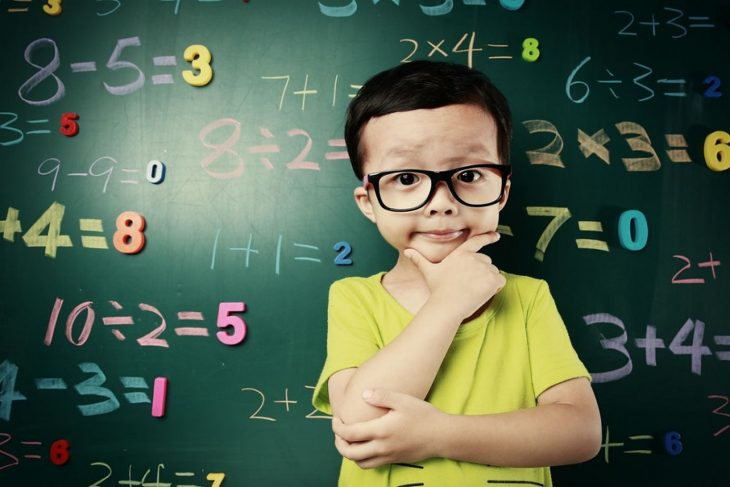## Latest Facts

### 14 Extraordinary Facts About Moto Guzzi V7 III Stone SKai

Modified & Updated: 08 Sep 2023Before diving into the specific addition facts, let’s establish a strong foundation by understanding the basic principles of addition. Addition is a mathematical operation that combines two or more numbers to find their total or sum. It is denoted by the “+” symbol. The numbers being added are called addends, and the result is known as the sum.

## The Number Line Method

A useful visual tool for understanding addition is the number line. A number line is a straight line that represents the continuum of numbers. To add two numbers using the number line method, start with the first addend and move to the right by the value of the second addend. The endpoint on the number line represents the sum of the two numbers.

## 0 + 10 = 10

Adding zero to any number results in that number itself. Therefore, when we add zero to ten, the sum is ten. This fact holds true for any number; adding zero doesn’t change the value of the other addend.

## 1 + 9 = 10

The sum of one and nine is ten. Understanding this fact is crucial, as it introduces the concept of number pairs that add up to ten. These pairs are referred to as number bonds and form the basis for further mathematical operations.

## 2 + 8 = 10

Another number pair that adds up to ten is two and eight. By recognizing and internalizing these number pairs, mental math becomes more efficient and accurate, enabling faster calculations.Image from Pinterest

## 3 + 7 = 10

Continuing the pattern, three and seven form another number pair that yields a sum of ten. Mastering these number bonds allows for greater fluency in addition, to paving the way for tackling more complex problems.

## 4 + 6 = 10

The number pair consisting of four and six also adds up to ten. It’s fascinating to see the symmetry and patterns that emerge in mathematics, and recognizing these patterns can make addition both enjoyable and intuitive.

## 5 + 5 = 10

Five and five make a perfect number bond of ten. This fact is particularly interesting since it showcases the concept of equality in addition. The two addends are equal, resulting in a balanced sum.

## 6 + 4 = 10

Reversing the order of the addends from Fact 5, we find that six and four also sum up to ten. This demonstrates the commutative property of addition, which states that the order of the addends does not affect the sum.

## 7 + 3 = 10

Seven and three forms yet another number pair that adds up to ten. By practicing these addition facts, you will build a strong foundation in basic arithmetic, facilitating future mathematical pursuits.

## 8 + 2 = 10

The number bond of eight and two yields a sum of ten. This fact underscores the concept of complementary numbers, where two numbers combine to form a whole.

## 9 + 1 = 10

Lastly, the number pair of nine and one adds up to ten. This fact completes the set of addition facts to ten and solidifies your understanding of basic addition.

## Conclusion

Congratulations! You have now explored the 10 addition facts to 10, mastering the fundamentals of addition. By understanding these number pairs and practicing mental math techniques, you will enhance your computational skills and lay a strong mathematical foundation. Remember to approach addition with curiosity and embrace the patterns and symmetry that exist within the world of numbers. So go ahead, continue your mathematical journey with confidence, and explore the countless possibilities that lie ahead.

### What are number bonds?

Number bonds are pairs of numbers that, when added together, yield a specific sum. In the case of addition to ten, the number bonds consist of pairs of numbers that add up to ten, such as 2 and 8, or 5 and 5.

### How can I help my child master addition to ten?

Encourage your child to practice mental math by using number bonds and the number line method. Incorporate fun activities and games that involve addition, and provide plenty of opportunities for hands-on practice.

### Why is it important to learn addition to ten?

Addition to ten forms the basis for more advanced mathematical concepts. It helps develop mental math skills, strengthens number sense, and facilitates problem-solving abilities.

### Are there any online resources or apps to practice addition skills?

Yes, there are numerous online resources and educational apps available that offer interactive games and exercises to reinforce addition skills. Some popular options include Khan Academy, Math Playground, and ABCmouse.

### How can I make learning addition enjoyable for my students?

Make use of manipulatives, such as counters or cubes, to provide a hands-on learning experience. Incorporate games, puzzles, and real-world examples to make the learning process engaging and relatable.